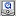- Computational Approach to Mathematical Sciences($B7W;;$K$h$k?tM}2J3X$NE83+(B), Video Archives - $B%W%m%8%'%/%H$N%[!<%(B(project home) ($BD{@5Ey$O$3$A$i$K7G<($5$l$k$3$H$b$"$j$^$9(B(errata might be posted here))  Lecture($B9V1i Title($B%?%$%H%k(B): Construction of high rank elliptic curves and related Diophantine problems Level: Research lecture$B7A<0(B(format): QuickTime($BF|K\8l(B) , QuickTime(English) (Version 7 or later) Source: AC2007 Abstract($BFbMF(B): By Mordell's theorem, the group of an elliptic curve over the rationals is the product of a finite subgroup consisting of all torsion points and$r \ge 0$copies of an infinite cyclic group. There are exactly 15 possible torsion groups, but little is known about which values of rank$r$are possible. The conjecture is that rank can be arbitrary large, but it seems to be very hard to find examples with large rank. The current record is an example of elliptic curve over$\mathbb{Q}$with rank$\geq 28$, found by Elkies in May 2006. There is even a stronger conjecture that for any of 15 possible torsion groups$T$we have$B(T)=\infty$, where$B(T)=\sup \{ {\rm rank}\,(E(\mathbb{Q})) \,:\, \mbox{torsion group of $E$ over $\mathbb{Q}$ is $T$} \}$. It follows from results of Montgomery and Atkin \& Morain that$B(T)\geq 1$for all admissible torsion groups$T$. We improved this result by showing that$B(T)\geq 3$for all$T$. In this talk, we will describe some recent improvements on lower bounds for$B(T)$. The information about current records for all admissible torsion groups can be found on my web page http://web.math.hr/~duje/tors/tors.html. Construction of high-rank curves in families of elliptic curves appears naturally in several Diophantine problems. We will present results related to elliptic curves induced by Diophantine triples, i.e. curves of the form$y^2=(ax+1)(bx+1)(cx+1)$, where$a,b,c$are non-zero rationals such that$ab+1$,$ac+1$and$bc+1$are perfect squares. We show that there are exactly four types of possible torsion groups, and construct curves with rank equal to$r$, for$r \le 9$. We will also consider arithmetic progressions consisting of integers which are solutions of a Pellian equation. In a recent joint paper with A.Peth\H{o} and P.Tadi\'{c}, we have constructed a seven-term arithmetic progression with the given property, and also several five-term arithmetic progressions which satisfy two different Pellian equations. These results are obtained by studying properties of a parametric family of elliptic curves. Message from the speaker: Keywords: serial=0051, type=research lecture License($B%i%$%;%s%9(B): No modification and free distribution. (creative commons, Attribution-NoDerivative works 2.1) Details #### Attachments (PDF) ### Lecture video ($B9V5A1GA|(B)

If you watch movies by streaming on Windows, please do not stop it by the "x" button. Your network connection might become very slow.
`